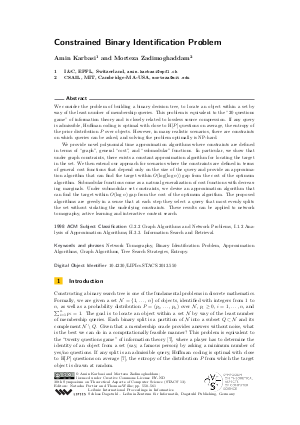Document# Constrained Binary Identification Problem## File

LIPIcs.STACS.2013.550.pdf
• Filesize: 0.56 MB
• 12 pages

## Cite As

Amin Karbasi and Morteza Zadimoghaddam. Constrained Binary Identification Problem. In 30th International Symposium on Theoretical Aspects of Computer Science (STACS 2013). Leibniz International Proceedings in Informatics (LIPIcs), Volume 20, pp. 550-561, Schloss Dagstuhl - Leibniz-Zentrum für Informatik (2013)
https://doi.org/10.4230/LIPIcs.STACS.2013.550

## Abstract

We consider the problem of building a binary decision tree, to locate an object within a set by way of the least number of membership queries. This problem is equivalent to the "20 questions game" of information theory and is closely related to lossless source compression. If any query is admissible, Huffman coding is optimal with close to H[P] questions on average, the entropy of the prior distribution P over objects. However, in many realistic scenarios, there are constraints on which queries can be asked, and solving the problem optimally is NP-hard. We provide novel polynomial time approximation algorithms where constraints are defined in terms of "graph", general "cost", and "submodular" functions. In particular, we show that under graph constraints, there exists a constant approximation algorithm for locating the target in the set. We then extend our approach for scenarios where the constraints are defined in terms of general cost functions that depend only on the size of the query and provide an approximation algorithm that can find the target within O(log(log n)) gap from the cost of the optimum algorithm. Submodular functions come as a natural generalization of cost functions with decreasing marginals. Under submodular set constraints, we devise an approximation algorithm that can find the target within O(log n) gap from the cost of the optimum algorithm. The proposed algorithms are greedy in a sense that at each step they select a query that most evenly splits the set without violating the underlying constraints. These results can be applied to network tomography, active learning and interactive content search.
##### Keywords
• Network Tomography
• Binary Identification Problem
• Approximation Algorithms
• Graph Algorithms
• Tree Search Strategies
• Entropy

## Metrics

• Access Statistics
• Total Accesses (updated on a weekly basis)
0